# An Overview of Dual Converters

Published  February 25, 2019   0
R
Author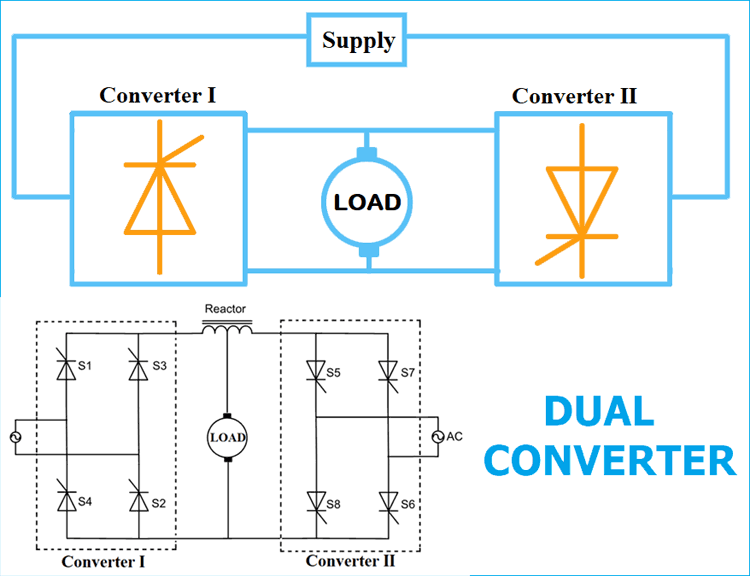In the previous tutorial we have seen how a Dual Power Supply Circuit is designed, now we learn about Dual Converters, which can convert AC to DC and DC to AC at the same time. As the name suggests Dual Converter has two converters, one converter works a rectifier (Converts AC to DC) and other converter works as an inverter (converts DC into AC). Both converters are connected back to back with a common load as shown in above picture. To learn more about Rectifier and Inverter, follow the links.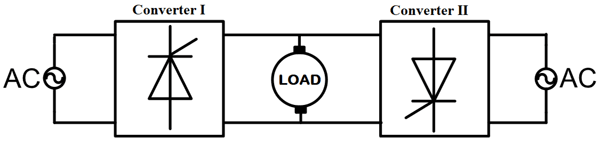Why we use the dual converter? If only one converter can supply the load, then why we use two converters? These questions may arise and you will get the answer in this article.

Here we have two converters connected back to back. Due to this type of connection, this device can be designed for four-quadrant operation. It means that both the load voltage and load current become reversible. How four-quadrant operation is possible in the dual converter? That we will see further in this article.

Generally, dual converters are used for the reversible DC drives or variable speed DC drives. It is used for high-power applications.

### Four Quadrant Operation in Dual Converter

First quadrant: voltage and current both positive.

Second quadrant: voltage is positive and current is negative.

Third quadrant: voltage and current both negative.

Fourth quadrant: voltage is negative and current is positive.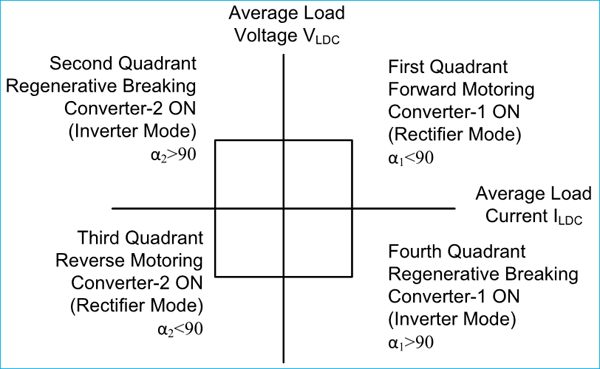Out of these two converters, the first converter works in two quadrants depending upon the value of firing angle α. This converter works as a rectifier when the value of α is less than 90˚. In this operation, the converter produces a positive average load voltage and load current, and operates in the first quadrant.

When the value of α is greater than 90˚, this converter works as an inverter. In this operation, the converter produces negative average output voltage and direction of current is not changed. That’s why the load current remains positive. In the first quadrant operation, the energy transfers from the source to the load and in the fourth quadrant operation, the energy transfers from the load to source.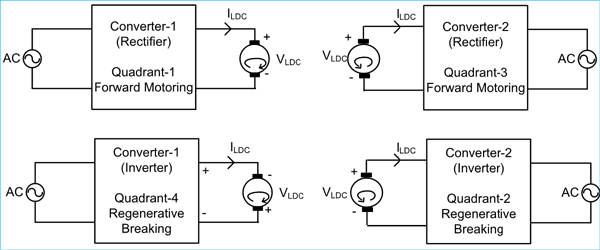Similarly, the second converter operates as a rectifier when firing angle α is less than 90˚ and it operates as an inverter when firing angle α is greater than 90˚. When this converter operates as a rectifier, the average output voltage and current both are negative. So, it operates in the third quadrant and power flow is from load to source. Here, the motor rotates in reverse direction. When this converter operates as an inverter, the average output voltage is positive and current is negative. So, it operates in the second quadrant and the power flow is from load to source.

When power flow is from load to source, the motor behaves like a generator and this makes regenerative breaking possible.

### Principle of the Dual Converter (Ideal Dual Converter)

To understand the principle of the dual converter, we assume that both converters are ideal. It means that they produce pure DC output voltage, there is no ripple at the output terminals. The simplified equivalent diagram of the dual converter is as shown in the below figure.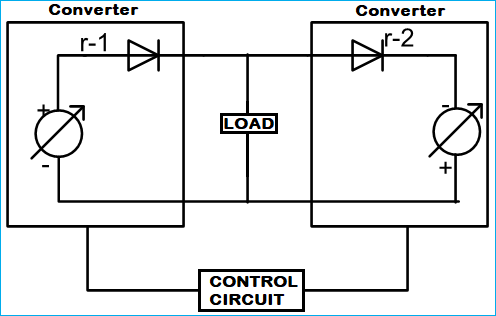In the above circuit diagram, the converter is assumed as a controllable DC voltage source and it is connected in series with the diode. The firing angle of the converters is regulated by a control circuit. So, the DC voltages of both converters are equal in magnitude and opposite in polarity. This makes possible to drive current in reverse direction through the load.

The converter operating as a rectifier is called a positive group converter and the other converter working as an inverter is called a negative group converter.

The average output voltage is a function of the firing angle. For single-phase inverter and three-phase inverter, the average output voltage is in the form of below equations.

```EDC1 = EmaxCos⍺1
EDC2 = EmaxCos⍺2```

Where α1 and α2 is the firing angle of converter-1 and converter-2 respectively.

For, single-phase dual converter,

`Emax = 2Em /π`

For, three-phase dual converter,

`Emax = 3√3Em /π`

For, ideal converter,

```EDC = EDC1 = -EDC2
EmaxCos⍺1 = -EmaxCos⍺2
Cos⍺1 = -Cos⍺2
Cos⍺1 = Cos (180⁰ - ⍺2)
⍺1 = 180⁰ - ⍺2
⍺1 + ⍺2= 180⁰```

As discussed above, the average output voltage is a function of the firing angle. It means for desired output voltage we need to control the firing angle. A firing angle control circuit can be used such that, when control signal Ec changes, firing angle α1 and α2 will change in such a way that it will satisfy below graph.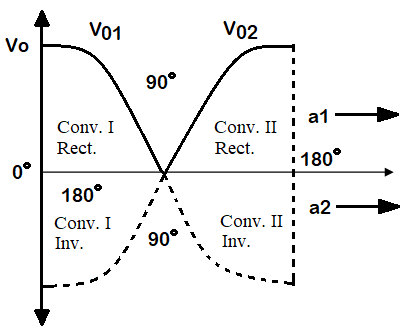### Practical Dual Converter

Practically we cannot assume both converters as an ideal converter. If the firing angle of converters are set in such a way that ⍺1 + ⍺2= 180⁰. In this condition, the average output voltage of both converters are same in mmagnitude but opposite in polarity. But due to ripple voltage, we cannot exactly get the same voltage. So, there are instantaneous voltage difference at the DC terminals of the two converters which produce enormous circulating current between the converters and that will flow through the load.

Therefore, in the practical dual converter, it is necessary to control the circulating current. There are two Modes to control the circulating current.

1) Operation without circulating current

2) Operation with circulating current

### 1) Dual Converter Operation without Circulating Current

In this type of dual converter, only one converter is in conduction and another converter is temporarily blocked. So, at a time one converter operates and the reactor is not required between the converters. At a particular instant, let say converter-1 acts as a rectifier and supplying the load current. At this instant, converter-2 is blocked by removing the firing angle. For inversion operation, converter-1 is blocked and converter-2 is supplying the load current.

The pulses to the converter-2 are applied after a delay time. The delay time is around 10 to 20 msec. Why we apply delay time between the change of operation? It ensures reliable operation of thyristors. If converter-2 trigger before the converter-1 has completely turned off, a large amount of circulating current will flow between converters.

There are many control schemes to generate a firing angle for circulating current free operation of the dual converter. These control schemes are designed to operate very sophisticated control systems.  Here, at a time only one converter is in conduction. Therefore, it is possible to use only one firing angle unit. A few basic schemes are listed below.

A) Converter selection by control signal polarity

B) Converter selection by load current polarity

C) Converter selection by both control voltage and load current

### 2) Dual Converter Operation with Circulating Current

In without circulating current converter, it requires very sophisticated control system and the load current is not continuous. To overcome these difficulties, there is a dual converter which can operate with the circulating current. A current limiting reactor is connected between the DC terminals of both converters. The firing angle of both converters is set in such a way that the minimum amount of circulating current flow through the reactor. As discussed in the ideal inverter, the circulating current is zero if ⍺1 + ⍺2= 180⁰.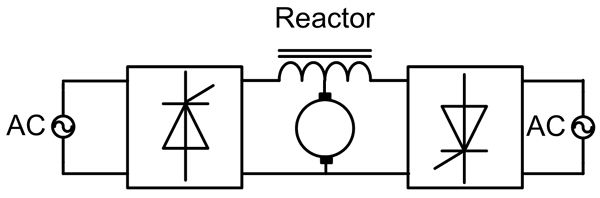Let say the firing angle of converter-1 is 60˚ then firing angle of converter-2 must be maintained at 120˚. In this operation, converter-1 will operate as a rectifier and converter-2 will operate as an inverter. Thus, in this type of operation, at a time both converters are in conducting state. If the load current is reversed, the converter which is operated as a rectifier is now operating as an inverter, while the converter which is operated as an inverter is now operating as a rectifier. In this scheme, both converters conduct at the same time. So, it requires two firing angle generator unit.

The advantage of this scheme is that we can get smooth operation of the converter at the time of inversion. Time response of the scheme is very fast. The normal delay period is 10 to 20 msec in the case of circulating current free operation is eliminated.

The disadvantage of this scheme is that, the size and cost of reactor high. Because of the circulating current, the power factor and efficiency are low. To handle the circulating current, the thyristors with high current ratings are required.

According to the type of load, single-phase and three-phase dual converters are used.

### 1) Single-Phase Dual Converter

The circuit diagram of the dual converter is shown in the below figure. A separately excited DC motor is used as a load. The DC terminals of both the converters are connected with the terminals of the armature winding. Here, two single-phase full converters are connected back to back. Both converters supply a common load.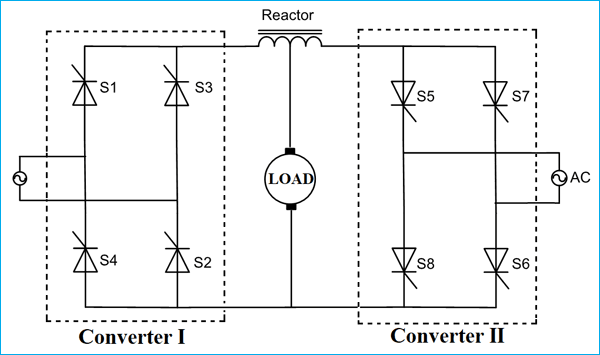The firing angle of converter-1 is α1 and α1 is less than 90˚. Hence, the converter-1 act as a rectifier. For positive half cycle (0 < t < π), thyristor S1 and S2 will conduct and for a negative half cycle (π < t < 2π), thyristor S3 and S4 will conduct. In this operation, output voltage and current both are positive. So, this operation is known as forward motoring operation and the converter works in the first quadrant.

The firing angle of converter-2 is 180 - α1 = α2 and α2 is greater than 90˚. So, converter-2 act as an inverter. In this operation, the load current remains in the same direction. The polarity of the output voltage is negative. Therefore, the converter works in the fourth quadrant. This operation is known as regenerative braking.

For reverse rotation of DC motor, converter-2 act as rectifier and converter-1 act as an inverter. The firing angle of converter-2 α2 is less than 90˚. The alternative voltage source supplies the load. In this operation, the load current is negative and the output average voltage is also negative. Therefore, the converter-2 works in the third quadrant. This operation is known as the reverse motoring.

In reverse operation, the firing angle of converter-1 is less than 90˚ and firing angle of converter-2 is greater than 90˚. So, in this operation, the load current is negative but the average output voltage is positive. So, the converter-2 works in the second quadrant. This operation is known as the reverse regenerative braking.

The waveform of the single-phase dual converter is as shown in the below figure.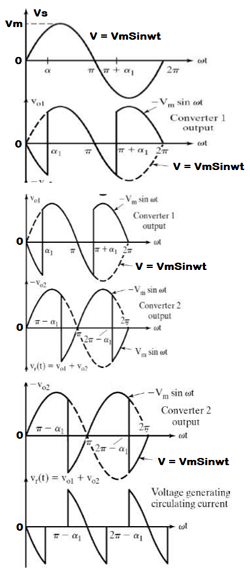### 2) Three-Phase Dual Converter

The circuit diagram of the three-phase dual converter is as shown in the below figure. Here, two three-phase converters are connected back to back. The principle of operation is the same as a single-phase dual converter.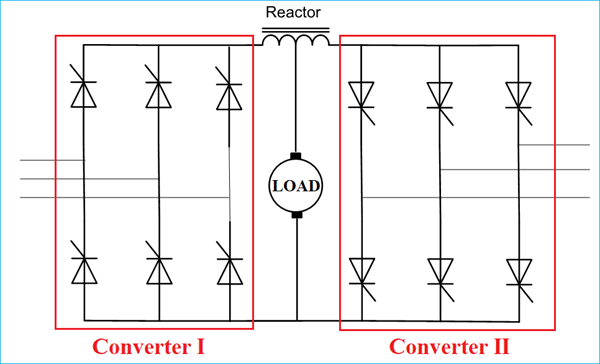So this is how Dual Converters are designed and as already told they are generally used to build reversible DC drives or variable speed DC drives in high-power applications.